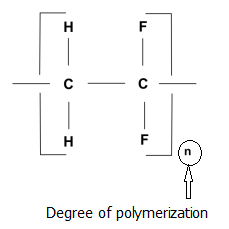# How to Calculate Degree of Polymerization

Polymerization is a chemical reaction that binds monomers to form large chains of polymers. A polymer will ultimately have repeating units since it binds multiple monomer units. This number of repeating units helps to determine physical and chemical properties of a polymer and vary depending on the polymer and its molecular weight. Hence, calculation of the number of repeating unit, or the degree of polymerization (DP) is very important in the polymer industry.

1. What is Degree of Polymerization?

2. How to Calculate Degree of Polymerization?

## What is Degree of Polymerization

The term degree of polymerization is defined as the number of repeating units in a polymer molecule. In some cases, this term is used to express the number of monomer units in an average polymer molecule. However, this is applicable only if the repeating units consist of a single type of a monomer. It is usually denoted as ‘n’ in the generalized formula -[ M ]n; where M is the repeating unit.## How to Calculate Degree of Polymerization

A polymer sample usually contains a distribution of chains with different degrees of polymerization. Therefore, an average value must be taken when determining the DP. The degree of polymerization can be calculated by using the following relationship if a molecular weight of a polymer molecule is known.

M= (DP) M0

M is the molecular weight of the polymer, DP is the degree of polymerization and the M0 is the formula weight of the repeating unit.

Ex: calculate the degree of polymerization of a sample of polyethylene [ (CH2-CH2)n], which has a molecular weight of 150,000 g/mol.

The molecular weight of a repeating unit, Mo= (12 x 2 + 1 x 4) g/ mol = 28 g/mol

DP = M/Mo

= 150,000 g/mol / 28 g/mol

= 5.35 x 103

The particular molecule contains 5.35 x 103 of repeat units.

When considering the molecular weight of a polymer for the above calculation, we usually take either the number average molecular weight (Mn) or weight average molecular weight (Mw).

### Formula to Calculate Number Average Molecular Weight

The number average molecular weight can be determined by,

Mn= Σ xi Mi

xi is the fraction of the total number of chains within each range, and Mi is the mean molecular weight of each size range of polymer chains.

### Formula to Calculate Weight Average Molecular Weight

The weight average molecular weight can be determined by,

Mw= Σ fi Mi

fi is the weight fraction of the polymer chains, and Mi is again the mean molecular weight of each range.

Reference:

Stuart, B. H. (2008).Polymer analysis (Vol. 30). John Wiley & Sons.

Rudin, A., & Choi, P. (2012).The elements of polymer science and engineering. Academic press.

Alger, M. (1996).Polymer science dictionary. Springer Science & Business Media.

Hannant, D. J. (1989). The Science and Engineering of Materials: By Donald R. Askeland. PWS, Boston, MA, USA, 1989. ISBN 0-534-91657-0. 876 pp.